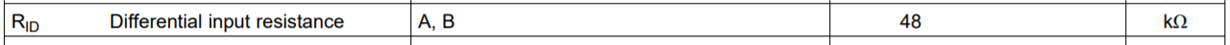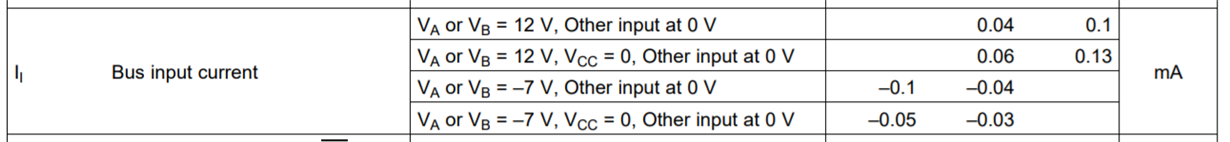• TI Thinks Resolved

# ISO3082: Input resistance

Part Number: ISO3082

Hi team,

I'm SO confused about the definition of input resistance. As you can see, in ISO3082's datasheet, it says the differential input resistance is 48kΩ. So what's the meaning of "Differential"? Does it mean that the input resistance is from bus A to bus B? I think it should be Bus A or Bus B to GND.However, it's much more easier to understand the input resistance in THVD1510's datasheet. It's highlighted that the input impedance is bus input impedance.So how to understand it?

Br,

Naizeng Wang

• Hi Naizeng,

Your initial assumption is correct. "Differential input resistance" refers to the the resistance between the A and B pins on the device. This is an important specification because it represents the resistive load presented by this device when it is added to the bus.The datasheet does not explicitly define input resistance between the A or B pins with respect to ground because this value varies with the applied voltage. Instead, we specify the bus input current given the Max and Min recommended operating voltages. If you require the input resistance with respect to ground, it can be calculated using this (lowest resistance encountered at Va or Vb = -7V).I hope this helps clarify the definition and purpose of differential input resistance. Let me know if you have any more questions.

Regards,
Eric

• In reply to Eric Schott1:

Hi Eric,

How to calculate the number of RS485 points using this differential input impedance?

Given the common impedance 96kΩ, I can conclude that the number is 32*96k/12k=256

But if the differential impedance is 48kΩ, I can't get the result.

So what's the relationship of common impedance and differential impedance??

Thanks

• In reply to Naizeng Wang:

Hi Naizeng,

I also see the discrepancy here. I will look into this further to see if this is the result of a typo or something I may be missing and get back to you on Monday .

Until then, I would advise that you base your calculations on the leakage current from the bus pins to ground (second figure I shared). An explanation on how to use this specification to determine the allowable number of loads on an RS-485 bus can be found in this technical article:
RS-485 basics: how to calculate unit loads and the maximum number of nodes on your network

Regards,
Eric

• In reply to Eric Schott1:

Hi Naizeng,

Thanks for your patience. I was able to confirm that the differential input resistance specification is a typo on this datasheet. This device does indeed have a 1/8th unit load allowing 256 nodes to be present on the bus. I will work on updating the datasheet values to reflect this. Thanks for bringing it to our attention.

Regards,
Eric